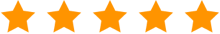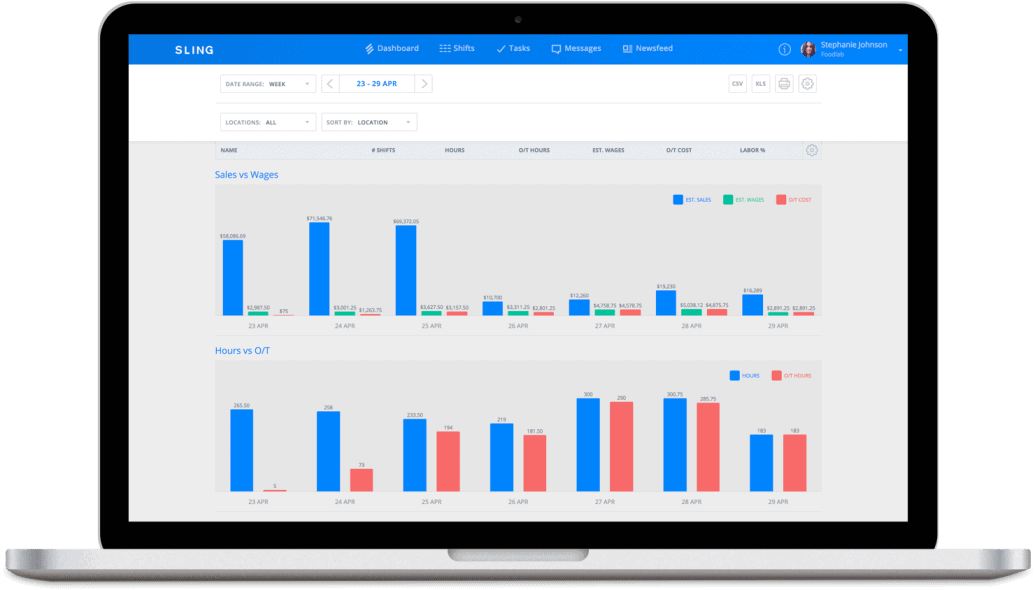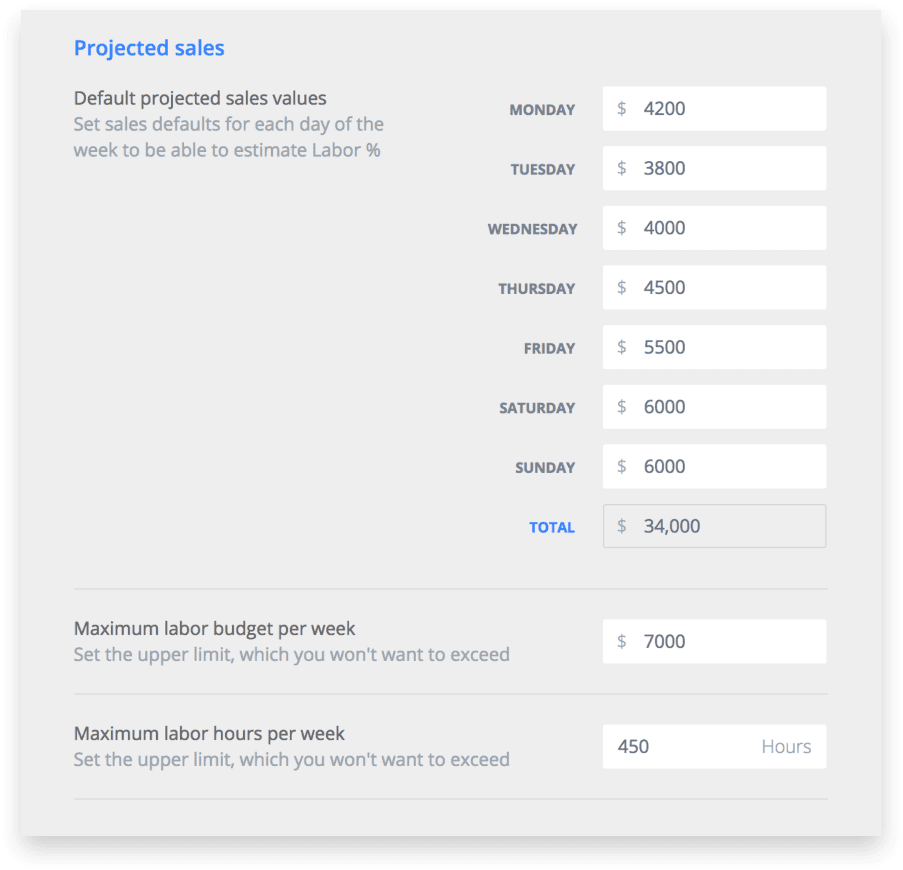"Sling allows businesses to schedule smarter instead of harder." - Bradley Knebel

## Contribution Margin Ratio: What It Is And How To Calculate ItContribution margin ratio is one of the most important business terms every manager needs to know but few actually do. Instead, they leave this number to their accountants and bookkeepers. In doing so, they lose sight of a valuable piece of their business.

Because to really understand your business, you have to control your contribution margin ratio.

In this article, the experts at Sling will help you understand contribution margin ratio better, show you how to calculate it, and reveal the best way to reduce this ratio to generate more profit.

## What Is Contribution Margin Ratio?Contribution margin ratio is the difference between your business’s sales (or revenue) and variable expenses for a given time period. As the name suggests, contribution margin ratio is expressed as a percentage.

Contribution margin ratio is useful for determining:

• Whether to lower your price (in a special pricing situation like a bulk buy)
• How changes in sales will impact your profits

The time period you choose to examine is irrelevant as long as sales and variable expenses are from the same dates.

Before we show you how to calculate contribution margin ratio, it’s vital to discuss the specific terms that make up the equation.

### Sales

Sales (a.k.a. total sales or revenue) is the monetary value of the goods or services sold by your business during a certain reporting period (e.g., quarterly or annually).

### Variable Expenses

Variable expenses are costs that change in conjunction with some other aspect of your business. Cost of materials purchased is a variable expense because it increases as sales increase or decreases as sales decrease.

Other variable expenses include:

• Labor
• Credit card fees
• Commissions
• Piece rate labor

When preparing to calculate contribution margin ratio, you will need to add together all of your variable expenses into one number.

## How Do You Calculate Contribution Margin Ratio?Calculating your business’s contribution margin ratio is not a difficult process. But you will need those two key pieces of data mentioned above:

• Total sales for a specific time period
• Total variable expenses for that same time period

The interesting thing about contribution margin ratio is that you can perform the calculation anytime to achieve a unique view into your business.

So, for example, you could calculate contribution margin ratio annually for a broad view into the impact of changes to sales, or calculate it on a single sale for a precise view into how your business is running.

### Contribution Margin Ratio Formula And Explanation

The formula for calculating contribution margin ratio is:

Contribution Margin Ratio = (Total Sales – Total Variable Expenses) / Total Sales

Let’s say your business sold \$2,000,000 in product during the first quarter of the year. Variable expenses for that same time period totaled \$500,000.

Substituting those numbers into the formula gives you:

Contribution Margin Ratio = (\$2,000,000 – \$500,000) / \$2,000,000

Working through the numbers gives you:

Contribution Margin Ratio = \$1,500,000 / \$2,000,000

Contribution Margin Ratio = 0.75

Contribution Margin Ratio = 75%

Think of the contribution margin ratio you calculate as the percentage of profit you achieved after variable expenses were paid. The higher the number the higher the profit.

### How To Use Contribution Margin Ratio With Other Numbers#### Net Profit Or Loss

Notice in the formula we used to calculate contribution margin ratio that we also calculated basic contribution margin as a dollar figure. This number is the amount of profit after variable expenses have been paid. We’ve highlighted it in the formula below:

Contribution Margin Ratio = (\$2,000,000 – \$500,000) / \$2,000,000

Contribution Margin Ratio = (\$1,500,000) / \$2,000,000

So in our example, your business made \$1,500,000 off sales of \$2,000,000.

Once you’ve calculated your contribution margin, use this number in conjunction with your total fixed expenses for the given time period to calculate net profit or net loss.

Say your business spent \$1,700,000 in fixed expenses on top of the \$500,000 in variable costs. The variable costs are already taken out of the \$1,500,000 contribution margin figure, so all you need to do is subtract fixed expenses to find net profit or loss. Here’s the formula:

Net Profit or Loss = Contribution Margin – Fixed Expenses

Net Profit or Loss = \$1,500,000 – \$1,700,000

Net Profit or Loss = -\$200,000

So, in this instance, your business has a net loss of \$200,000.

#### Break-Even Threshold

Once you know that you have a net loss on your hands, you can use contribution margin ratio to figure out what you need to do to break even. You could, of course, reduce your fixed expenses by \$200,000. But you could also increase sales by \$200,000 without increasing variable expenses.

## Increase Contribution Margin Ratio By Controlling Labor Costs

If you want to reduce your variable expenses — and thereby increase your contribution margin ratio — start by controlling labor costs.

Labor costs make up a large percentage of your business’s variable expenses, so it’s the ideal place to start making changes. And the quickest way to make the needed changes is to use a scheduling and labor management tool like Sling.With the help of advanced artificial intelligence, Sling lets you set projected labor costs before you schedule your employees so you know what the wage ceiling will be before putting names to paper. Once those values are set, you can create the perfect schedule the first time through…without going over your labor budget.

See in real-time what each shift will cost your business and adjust the expenses accordingly.That can help transform your labor costs from a variable expense to a fixed expense and allow you to keep those expenses under tighter control. As a result, your variable expenses will go down and your contribution margin ratio will go up. That means more profit for your business.

For more free resources to help you manage your business better, organize and schedule your team, and track and calculate labor costs, visit GetSling.com today.

#### Get started today

Schedule faster, communicate better, get things done.